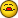Code For Copy Copies Twice

P

PHisaw

Hi,

Thanks to all who have helped with code for my workbook, I have managed to
piece it all together to perform several task with the click of a button, but
have two small glitches I hope someone can help resolve.

The last bit of code " 'copy summary from main worksheet" should copy
w1:am75 and place in same location on each of the worksheets listed. It will
do this but also copies w34:am75 and places it underneath the first copy of
w1:am75. The second portion that is copied has lines inserted at each place
a total line is inserted from code listed above. I tried several ways of
rearranging the code thinking it was something in the looping process and
nothing seems to correct it.

If anyone can please take a look and tell me what is going wrong with this
and possibly clean up code as needed, I would really appreciate the
assistance. Also, how do I make the highlight for the total rows that are
found in cols B & C extend left to A & B. I would like for it to cover the
section A, but this is the only code I could find that would work.

Sub Total_Bookings_WorksheetsTest2()

Dim ws As Worksheet
Dim rng As Range

For Each ws In Worksheets

Select Case ws.Name

'All sheet names listed in the case statement
'will be processed. Change the names I have
'additional sheet names separated by commas.
Case "Bk01-09", "Bk02-09"

ws.Select
'Sort selected worksheets
Range("A1900").Select
Selection.Sort Key1:=Range("c2"), _
Order1:=xlAscending, _
Key2:=Range("A2"), Order2:=xlAscending, _
Key2:=Range("b2"), Order2:=xlAscending, _

On Error Resume Next
'Following line references active sheet so
'do not nest inside the With/End With
Set rng = Range(Range("j2"), _
Cells(2, Columns.Count).End(xlToLeft))
On Error GoTo 0

If Not rng Is Nothing Then

With ws
'Subtotal selected sheets
.Range("j2").Subtotal _
GroupBy:=3, _
Function:=xlSum, _
TotalList:=Array(10, 11, 12), _
Replace:=False, _
PageBreaks:=False, _
SummaryBelowData:=True

.Range("j2").Subtotal _
GroupBy:=1, _
Function:=xlSum, _
TotalList:=Array(10, 11, 12), _
Replace:=False, _
PageBreaks:=False, _
SummaryBelowData:=True

.Range("j2").Subtotal _
GroupBy:=2, _
Function:=xlSum, _
TotalList:=Array(10, 11, 12), _
Replace:=False, _
PageBreaks:=False, _
SummaryBelowData:=True

'Format area for summary formulas from main sheet
.Range("w2:am75").NumberFormat = "\$#,##0.00;(\$#,##0.00)"
.Range("w2:am75").Font.Size = 8

End With

End If
'Bold and insert row at "total" rows
Dim LastRow As Long
Dim r As Long
'Following code references active sheet so
'do not nest inside the With/End With
LastRow = Range("G" & Rows.Count).End(xlUp).Row
For r = LastRow To 2 Step -1
If InStr(1, Cells(r, 1).Value, "Total") > 0 Or _
InStr(1, Cells(r, 2).Value, "Total") > 0 Or _
InStr(1, Cells(r, 3).Value, "Total") > 0 Or _
InStr(1, Cells(r, 4).Value, "Total") > 0 Then
Range(Cells(r, 1), Cells(r, 16)).Font.Bold = True
ActiveSheet.Rows(r + 1).EntireRow.Insert
End If
Next

'Highlight "total" rows
Dim rngFound As Range

'Search slsp (Col A) for Total rows & highlight
Set rngFound = Columns("A").Find(What:="total", _
LookAt:=xlPart, _
LookIn:=xlValues, _
MatchCase:=False)
If Not rngFound Is Nothing Then
Do
rngFound.Resize(, 16).Interior.ColorIndex = 17
Set rngFound = Columns("A").FindNext(rngFound)
End If

'Search Class (Col B) for Total rows & highlight
Set rngFound = Columns("B").Find(What:="total", _
LookAt:=xlPart, _
LookIn:=xlValues, _
MatchCase:=False)
If Not rngFound Is Nothing Then
Do
rngFound.Resize(, 15).Interior.ColorIndex = 6
Set rngFound = Columns("B").FindNext(rngFound)
End If

'Search Dept (Col C) for Total rows & highlight
Set rngFound = Columns("c").Find(What:="total", _
LookAt:=xlPart, _
LookIn:=xlValues, _
MatchCase:=False)
If Not rngFound Is Nothing Then
Do
rngFound.Resize(, 14).Interior.ColorIndex = 23
Set rngFound = Columns("c").FindNext(rngFound)
End If
End Select 'End of Case

'copy summary section from main worksheet
Dim wsrng As Range
Dim myarray()
Dim i As Long
Set wsrng = Worksheets("Bookings").Range("w1:AM75")

myarray = Array("Bk01-09", "Bk02-09")

For i = LBound(myarray) To UBound(myarray)

Worksheets(myarray(i)).Range("w1:AM75").Formula = wsrng.Formula

'replace formula with .value if you want to copy cell values
Next

Next ws

End Sub

Again, I would really appreciate the help.
Phisaw

J

JLGWhiz

There must be some stray code out there somewhere, or my eyes are worse than
I thought. The code you posted does not refer to the second set of data
that you said it is pasting below the desired range. The way to find out
for sure what is happening is to step through the code by using the F8
function key. Open the VBE and place the insertion point (cursor) within
the procedure code somewhere. Then press F8 to start the code execution,
the yellow highlight will show you which line is next to execute. If you
diminishe the size of the VBE screed and manually size it you can position
it over the worksheets so that you can see when changes in the data occur as
you step through. Notice the title bar at the top left and it will display
the active sheets. Also, the Project window in the VBE will indicate which
module, shee4t or form is executing code by shadowing that object. You
should be able to isolate the problem with this debugging technique.

P

PHisaw

Thanks Joel and JLGWhiz for the tip to step thru code. I placed the cursor
at this line of code:

ws.select

(in the first part of code after the case names of worksheets) and selected
"Run To Cursor". The section of the main worksheet (that is copied with the
last section of code) was copied at that point. After totaling and inserting
lines at total rows it made the section become spaced out with rows between
each making it longer on the sheet than the original. Then when the code ran
thru, the last section copied it again placing it on top of what was there
causing me to only see a portion.

When I remove the code at the bottom, it is not copied at this line.

Can either of you tell me why it is copying the section at this line of code
when I have the last portion included and how I should rearrange the code to
process it in order?

Thanks again for all your help.
Phisaw

P